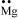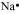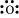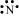Select Chapter Topics:# In the option are given sequences of increasing bond order for the following species. The correct option is : ${\mathrm{O}}_{2}$ $,$ ${\mathrm{N}}_{2}$ $,$ ${\mathrm{O}}_{2}^{+}$ $\mathrm{and}$ ${\mathrm{O}}_{2}^{-}$ is - $1.$ ${\mathrm{O}}_{2}$ $>$ ${\mathrm{N}}_{2}$ $>$ ${\mathrm{O}}_{2}^{+}$ $>$ ${\mathrm{O}}_{2}^{-}$$2.$ ${\mathrm{O}}_{2}^{-}$ $>$ ${\mathrm{O}}_{2}$ $>$ ${\mathrm{O}}_{2}^{}$ $+$ $>$ ${\mathrm{N}}_{2}$$3.$ ${\mathrm{N}}_{2}$ $>$ ${\mathrm{O}}_{2}^{+}$ $>{\mathrm{O}}_{2}^{}$ $>{\mathrm{O}}_{2}^{-}$$4.$ ${\mathrm{O}}_{2}^{-}$ $>$ ${\mathrm{O}}_{2}^{+}$ $>$ ${\mathrm{O}}_{2}$ $>$ ${\mathrm{N}}_{2}$Subtopic:  M.O.T |
85%
From NCERT
To view explanation, please take trial in the course.
NEET 2023 - Target Batch - Aryan Raj Singh
Hints
To view explanation, please take trial in the course.
NEET 2023 - Target Batch - Aryan Raj Singh

${\mathrm{AlCl}}_{3}$ $+$ ${\mathrm{Cl}}^{-}$ $\to$ ${\mathrm{AlCl}}_{4}^{-}$

The change in the hybridization of the Al atom in the above reaction is-
1. $$s p^{2} \text { to } s p^{3}$$
2. $$\mathrm{sp}^{3} \text { to } s p^{2}$$
3. $$\mathrm{sp}^{3} \text { to } \mathrm{dsp}{ }^{2}$$
4. $$\operatorname{sp}^{2} \text { to } d s p^{2}$$Subtopic:  Hybridisation |
83%
From NCERT
To view explanation, please take trial in the course.
NEET 2023 - Target Batch - Aryan Raj Singh
Hints
To view explanation, please take trial in the course.
NEET 2023 - Target Batch - Aryan Raj Singh

Considering the X-axis as the internuclear axis, a sigma bond will not be formed in:

$1.$ $1\mathrm{s}$ $\mathrm{and}$ $2\mathrm{s}$
$2.$ $1\mathrm{s}$ $\mathrm{and}$ $2{\mathrm{p}}_{\mathrm{x}}$
$3.$ $2{\mathrm{p}}_{\mathrm{y}}$ $\mathrm{and}$ $2{\mathrm{p}}_{\mathrm{y}}$
$4.$ $1\mathrm{s}$ $\mathrm{and}$ $1\mathrm{s}$Subtopic:  Hybridisation |
77%
From NCERT
To view explanation, please take trial in the course.
NEET 2023 - Target Batch - Aryan Raj Singh
Hints
To view explanation, please take trial in the course.
NEET 2023 - Target Batch - Aryan Raj Singh

The plus and negative sign of the orbitals mean:

1. Wave function.

2. Probability density.

3. Quantum number of orbitals.

4. Frequency of orbitals.Subtopic:  M.O.T |
63%
From NCERT
To view explanation, please take trial in the course.
NEET 2023 - Target Batch - Aryan Raj Singh
Hints
To view explanation, please take trial in the course.
NEET 2023 - Target Batch - Aryan Raj Singh

The relative order of stability of the following species: ${\mathrm{O}}_{2}$ $,$ ${\mathrm{O}}_{2}^{}$ $+$ $,$ ${\mathrm{O}}_{2}^{-}$ $\mathrm{and}$ ${\mathrm{O}}_{2}^{2-}$  is -

$1.$ ${\mathrm{O}}_{2}^{+}$ $>$ ${\mathrm{O}}_{2}>$ ${\mathrm{O}}_{2}^{-}$ $>$ ${\mathrm{O}}_{2}^{2-}$ 
$2.$ ${\mathrm{O}}_{2}^{2-}>{\mathrm{O}}_{2}$ $>$ ${\mathrm{O}}_{2}^{-}$ $>$ ${\mathrm{O}}_{2}^{+}$
$3.$ ${\mathrm{O}}_{2}$ $>$ ${\mathrm{O}}_{2}^{+}$  $>$ ${\mathrm{O}}_{2}^{-}$ $>$ ${\mathrm{O}}_{2}^{2-}$
$4.$ ${\mathrm{O}}_{2}$ $>$ ${\mathrm{O}}_{2}^{2-}$ $>{\mathrm{O}}_{2}^{-}$ $>$ ${\mathrm{O}}_{2}^{+}$Subtopic:  M.O.T |
81%
From NCERT
To view explanation, please take trial in the course.
NEET 2023 - Target Batch - Aryan Raj Singh
Hints
To view explanation, please take trial in the course.
NEET 2023 - Target Batch - Aryan Raj Singh

The incorrect statement about NH3 and H2O is -

1. The bond angle in NH3 is less than in H2O.

2. Both have distorted tetrahedral geometries.

3. The bond angle in H2O is less than in NH3.

4. Both are sp3 hybridized.Subtopic:  Hybridisation |
69%
From NCERT
To view explanation, please take trial in the course.
NEET 2023 - Target Batch - Aryan Raj Singh
Hints
To view explanation, please take trial in the course.
NEET 2023 - Target Batch - Aryan Raj Singh

The shape of  AsF5 is -

1. Pentagonal bipyramidal.

2. Trigonal bipyramidal.

3. Distorted tetrahedral.

4. Square pyramidal.Subtopic:  V.S.E.P.R & V.B.T |
73%
From NCERT
To view explanation, please take trial in the course.
NEET 2023 - Target Batch - Aryan Raj Singh
Hints
To view explanation, please take trial in the course.
NEET 2023 - Target Batch - Aryan Raj Singh

The reason of an atom behind the formation of a chemical bond is -

1. To increase the size.

2. To decrease size.

3. To attain stability.

4. None of the above.Subtopic:  Covalent Bond |
92%
From NCERT
To view explanation, please take trial in the course.
NEET 2023 - Target Batch - Aryan Raj Singh
Hints
To view explanation, please take trial in the course.
NEET 2023 - Target Batch - Aryan Raj Singh

The Lewis dot symbols for atoms of the elements Mg, Na, O, and N, respectively, would be:

 1234Subtopic:  Octate, Isoelectronic Species, Lewis Structure & Formal Charge |
84%
From NCERT
To view explanation, please take trial in the course.
NEET 2023 - Target Batch - Aryan Raj Singh
Hints
To view explanation, please take trial in the course.
NEET 2023 - Target Batch - Aryan Raj Singh

Lewis symbols for the atoms and ions: S and S2–; Al and Al3+; H and H respectively, would be:

 1${\left[\mathbf{:}\underset{\mathbf{.}\mathbf{.}}{\overset{\mathbf{.}\mathbf{.}}{\mathrm{S}}}\mathbf{:}\right]}^{2-}$ ${\left[\mathrm{Al}\right]}^{3+}$2${\left[\mathbf{:}\underset{\mathbf{.}\mathbf{.}}{\overset{\mathbf{.}\mathbf{.}}{\mathrm{S}}}\mathbf{:}\right]}^{2-}$${\left[\mathrm{Al}\right]}^{3+}$3 ${\left[\mathbf{:}\underset{\mathbf{.}\mathbf{.}}{\overset{\mathbf{.}\mathbf{.}}{\mathrm{S}}}\mathbf{:}\right]}^{2-}$${\left[\mathrm{Al}\right]}^{3+}$4${\left[\mathbf{:}\underset{\mathbf{.}\mathbf{.}}{\overset{\mathbf{.}\mathbf{.}}{\mathrm{S}}}\mathbf{:}\right]}^{2-}$${\left[\mathrm{Al}\right]}^{3+}$Subtopic:  Octate, Isoelectronic Species, Lewis Structure & Formal Charge |
79%
From NCERT
To view explanation, please take trial in the course.
NEET 2023 - Target Batch - Aryan Raj Singh
Hints